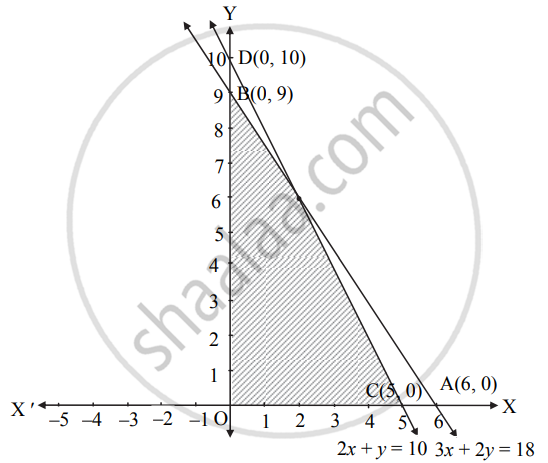Advertisement Remove all ads

# Find the feasible solution of the following inequation: 3x + 2y ≤ 18, 2x + y ≤ 10, x ≥ 0, y ≥ 0 - Mathematics and Statistics

Chart
Graph

Find the feasible solution of the following inequation:

3x + 2y ≤ 18, 2x + y ≤ 10, x ≥ 0, y ≥ 0

Advertisement Remove all ads

#### Solution

 Given inequalities 3x + 2y ≤ 18 2x + y ≤ 10 Corresponding equalities 3x + 2y = 18 2x + y = 10 Intersection of line with X-axis A(6, 0) C(5, 0) Intersection of line with Y-axis B(0, 9) D(0, 10) Origin test 3(0) + 2(0) ≤ 18 i.e., 0 ≤ 18 which is true 2(0) + 0 ≤ 10 i.e., 0 ≤ 10 which is true Region Origin side of the line Origin side of the line

x ≥ 0, y ≥ 0 represent 1st quadrant.

The shaded portion represents the feasible solution.Concept: Linear Programming Problem (L.P.P.)
Is there an error in this question or solution?
Advertisement Remove all ads

#### APPEARS IN

Advertisement Remove all ads
Advertisement Remove all ads
Share
Notifications

View all notifications

Forgot password?